# What is a Weber Fraction?

(Difference between revisions)
 Revision as of 01:57, 2 April 2011 (view source)Panamath (Talk | contribs) (→The Weber Fraction as a Scaling Ratio)← Older edit Revision as of 03:55, 2 April 2011 (view source)Panamath (Talk | contribs) Newer edit → Line 1: Line 1: + Because the site is currently under development, Panamath is still collecting all of the necessary citations for this wiki page. As such, no references will be provided until this process is complete. == Model Representations of the ANS == == Model Representations of the ANS ==

## Revision as of 03:55, 2 April 2011

Because the site is currently under development, Panamath is still collecting all of the necessary citations for this wiki page. As such, no references will be provided until this process is complete.

## Model Representations of the ANS

In modeling performance on tasks that engage the ANS, it is necessary first to specify a model for the underlying approximate number representations. It is generally agreed that each numerosity is mentally represented by a distribution of activation on an internal “number line.” These distributions are inherently “noisy” and do not represent number exactly or discretely. This means that there is some error each time they represent a number; and this error can be thought of as a spread of activation around the number being represented.

## The Mental Number Line

The mental number line is often modeled as having linearly increasing means and linearly increasing standard deviation. In such a format, the representation for e.g., cardinality seven is a probability density function that has its mean at 7 on the mental number line and a smooth degradation to either side of 7 such that 6 and 8 on the mental number line are also highly activated by instances of seven in the world. In Figure 1a I have drawn idealized curves which represent the ANS representations for numerosities 4-10 for an individual with Weber fraction = .125. You can think of these curves as representing the amount of activity generated in the mind by a particular array of items in the world with a different bump for each numerosity you might experience (e.g., 4 balls, 5 houses, 6 blue dots, etc). Rather than activating a single discrete value (e.g., 6) the curves are meant to indicate that a range of activity is present each time an array of (e.g., 6) items is presented. That is, an array of e.g., six items will greatly activate the ANS numerosity representation of 6, but because these representations are noisy this array will also activate representations of 5 and 7 etc with the amount of activation centered on 6 and gradually decreasing to either side of 6.

## Neuronal Associations of the Mental Number Line

The bell-shaped representations of number depicted in Figure 1a are more than just a theoretical construct; “bumps” like these have been observed in neuronal recordings of the cortex of awake behaving monkeys as they engage in numerical discrimination tasks (e.g., shown an array of six dots, neurons that are preferentially tuned to representing 6 are most highly activated, while neurons tuned to 5 and 7 are also fairly active, and those tuned to 4 and 8 are active above their resting state but less active than those for 5, 6, and 7. These neurons are found in the monkey brain in the same region of cortex that has been found to support approximate number representations in human subjects. This type of spreading, “noisy” activation is common throughout the cortex and is not specific to representing approximate number. Rather, approximate number representations obey principles that operate quite generally throughout the mind/brain.

## Interpreting the Gaussian Curves

The bell-shaped representations of number depicted in Figure 1a are more than just a theoretical construct; “bumps” like these have been observed in neuronal recordings of the cortex of awake behaving monkeys as they engage in numerical discrimination tasks (e.g., shown an array of six dots, neurons that are preferentially tuned to representing 6 are most highly activated, while neurons tuned to 5 and 7 are also fairly active, and those tuned to 4 and 8 are active above their resting state but less active than those for 5, 6, and 7. These neurons are found in the monkey brain in the same region of cortex that has been found to support approximate number representations in human subjects. This type of spreading, “noisy”, activation is common throughout the cortex and is not specific to representing approximate number. Rather, approximate number representations obey principles that operate quite generally throughout the mind/brain.

When trying to discriminate one numerosity from another using the Gaussian representations in Figure 1a, the more overlap there is between the two Gaussians being compared the less accurately they can be discriminated.

Ratios that are closer to 1, where the two numbers being compared are close (e.g., 9 versus 10), give rise to Gaussians with greater overlap resulting in poorer discrimination (i.e., “ratio-dependent performance”). The ratio is calculated with this formula, where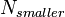$N_{smaller} \,$ is the smaller number and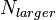$N_{larger} \,$ is the larger number, respectively:$Ratio = \frac{{N_{larger}}}{N_{smaller}} \,$

Visually, the curve for 5 in Figure 1a looks clearly different in shape from the curve for 4 (e.g., curve 4 is higher and skinnier than curve 5); and discriminating 4 from 5 is fairly easy. As you increase in number (i.e., move to the right in Figure 1a), the curves become more and more similar looking (e.g., is curve 9 higher and skinnier than curve 10?); and discrimination becomes harder.

But, it is not simply that larger numbers are harder to discriminate across the board. For example, performance at discriminating 16 from 20 (not shown) will be identical to performance discriminating 4 from 5 as these pairs differ by the same ratio (i.e., 5 / 4 = 1.125 = 20 / 16); and the curves representing these numbers overlap in the ANS such that the representation of 4 and 5 overlap in area to the same extent that 16 overlaps with 20 (i.e., although 16 and 20 each activate very wide curves with large standard deviations, these curves are far enough apart on the mental number line that their overlap is the same amount of area as the overlap between 5 and 4, i.e., they have the same discriminability). This is ratio-dependent performance.

## Tasks That Give Rise to the Gaussian Curves

The Gaussian curves in Figure 1a are depictions of the mental representations of 4-10 in the ANS. Similar looking curves can be generated by asking subjects to make rapid responses that engage the ANS, such as asking subjects to press a button 9 times as quickly as possible while saying the word “the” repeatedly to disrupt explicit counting. In such tasks, the resulting curves, generated over many trials, represent the number of times the subject pressed the button when asked to press it e.g., 9 times. Because the subject can’t count verbally and exactly while saying “the”, they tend to rely on their ANS to tell them when they have reached the requested number of taps.

When this is the case, the variance in the number of taps across trials is the result of the noisiness of the underlying ANS representations and so can be thought of as another method for determining what the underlying Gaussian representations are. That is, if starting to tap and ending tapping etc did not contribute additional noise to the number of taps (i.e., if the ANS sense of how many taps had been made were the only source of over and under tapping) then the standard deviation of the number of taps for e.g., 9 across trials would be identical to the standard deviation of the underlying ANS number representation of e.g., 9.

When attempting to visualize what the noisy representations of the ANS are like, one can think of the Gaussian activations depicted in Figure 1a, and these representations affect performance in a variety of tasks including discriminating one number for another (e.g., 5 versus 4) and generating number-relevant behaviors (e.g., tapping 9 times).

## Numerical Discrimination in the ANS

To understand how numerical discrimination is possible in the ANS, consider the task of briefly presenting a subject with two arrays, e.g., 5 yellow dots and 6 blue dots, and asking the subject to determine which array is greater in number (Figure 2a). The 5 yellow dots will activate the ANS curve representation of 5 and the 6 blue dots will activate the ANS curve representation of 6 (assume that the subject uses attention to select which dots to send to the ANS for enumerating and then stores and compares those numerosity representations bound to their respective colors) (Figure 2a-b).

## ANS Modeling and Subtraction

An intuitive way to think about ordinal comparison within the ANS is to liken it to a subtraction; this will be mathematically equivalent to other ways of making an ordinal judgment within the ANS and my use of subtraction here should be thought of as one illustration among several mathematically equivalent illustrations.

Imagine that an operation within the ANS subtracts the smaller (i.e., five-yellow) representation from the larger (i.e., six-blue) representation (Figure 2b). Because the 5 and 6 representations are Gaussian curves, this subtraction results in a new Gaussian representation of the difference which is a Gaussian curve on the mental number line that has a mean of 1 (6 − 5 = 1) and a standard deviation of: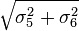$\sqrt{{{\sigma}_5^2 + {\sigma}_6^2}} \,$

In Figure 2c (i.e., when subtracting one Gaussian random variable from another (i.e.,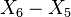$X_6 - X_5 \,$), the result is a new Gaussian random variable with the mean at the difference (6 – 5 = 1) and a variance that adds the variances of the original variables: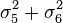${\sigma}_5^2 + {\sigma}_6^2 \,$. This results in a Gaussian curve that is centered on 1, but that extends to both the left and right of 0 (Figure 2c).

One can think of 0 as the demarcation line separating evidence “for” and “against” in that the area under the curve to the right of 0 is the portion of the resulting representation that correctly indicates that six is greater than five while the area under the curve to the left of 0 is the portion of the resulting representation that incorrectly indicates that five is greater than six. This area to the left of 0 results from the overlap between the original Gaussian representations, five and six, that were being discriminated in which some of the area of five-yellow is to the right (i.e., greater than) some of the area of six-blue (Figure 2b).

## Interpreting Gaussian Overlap: Weber's Law

One can think of 0 as the demarcation line separating evidence “for” and “against” in that the area under the curve to the right of 0 is the portion of the resulting representation that correctly indicates that six is greater than five while the area under the curve to the left of 0 is the portion of the resulting representation that incorrectly indicates that five is greater than six. This area to the left of 0 results from the overlap between the original Gaussian representations, five and six, that were being discriminated in which some of the area of five-yellow is to the right (i.e., greater than) some of the area of six-blue (Figure 2b).

Another method would rely on assessing the total evidence for blue and the total evidence for yellow. Either of these ways of making a decision will have the result that, on a particular trial, the probability of the subject getting the trial correct will depend on the relative area under the curve to the left and right of 0 which is itself determined by the amount of overlap between the original Gaussian representations for the numerosities being compared (i.e., five and six).

The more overlap there is between the two Gaussian representations being compared, the less accurately they can be discriminated. Consider comparing a subject’s performance on a 5 dots versus 6 dots trial to a trial involving 9 versus 10 dots. Using the curves in Figure 1a as a guide, we see that the overlapping area for the curves representing 5 and 6 is less than the overlapping area for the curves representing 9 and 10, because the curves flatten and spread as numerosity increases. This means that it will be easier for the subject to tell the difference between 5 and 6 than between 9 and 10, i.e., the resulting Gaussian for the subtraction will have more area to the right of 0 for the subtraction of 5 from 6 than for the subtraction of 9 from 10.

Across multiple trials the subject would give more correct responses on the 5 versus 6 trials than the 9 versus 10 trials owing to the greater evidence for 6 being larger than 5 (i.e., more area to the right of 0 in the subtraction, Figure 2c). The linear increase in the standard deviation of the curves representing the numerosites along the mental number line results in ratio-dependent performance whereby the discriminability of two numerosities increases as the ratio between them (e.g., biggernumber / smallernumber) increases. This is Weber’s law.

How rapidly performance rises from chance (50%) to near-asymptotic performance (100%) in this kind of dot numerosity discrimination task is controlled by the subject’s Weber fraction (w).

## The Weber Fraction: Overview

The Weber fraction indexes the amount of spread in the subject’s ANS number representations and therefore the overlap between any two numbers as a function of ratio (described in a succeeding section). The precision of the ANS varies across individuals with some people having a smaller Weber fraction (i.e., better performance and sharper Gaussian curves) and others having a larger Weber fraction (i.e., poorer performance owing to wider noisier Gaussian curves).

Numerical discrimination (e.g., determining which color, blue or yellow, has more dots in an array of dots flashed to quickly for explicit counting) is possible in the ANS through a process that attempts to determine which of the two resulting curves (e.g., five-yellow or six-blue) is further to the right on the mental number line. The fullness of these noisy curves is used to make this decision (and not just the mode, or mean or some other metric) and successful discrimination thereby depends on the amount of overlap between the two activated curves (i.e., ratio-dependent performance).

The amount of overlap is indexed by a subject’s Weber fraction (w) with a larger Weber fraction indicating more noise, more overlap, and thereby worse discrimination performance. This model has been found to provide an accurate fit to data from rats, pigeons, and humans of all ages.

## Relationship to the ANS

What does a Weber fraction (w) tell us about a subject’s Approximate Number System (ANS) representations? A relationship exists between the Weber fraction (w), the standard deviations of the underlying Gaussian numerosity representations, and the discriminability of any two numbers; and a Weber fraction can be thought of in at least three ways, which sometimes leads to confusions.

These are: 1) the fraction by which a stimulus with numerosity n would need to be increased in order for a subject to detect this change and its direction on 50% of the trials (aka the difference threshold, aka the Just Noticeable Difference, J.N.D.), 2) the midpoint between subjective equality of two numbers and asymptotic performance in discrimination, and 3) the constant that describes the standard deviation of all of the numerosity representations on the mental number line. Option 3 is perhaps the least discussed in the psychophysics literature - it is rarely taught in psychophysics courses - but I will suggest that option 3 is the most productive way to understand the Weber fraction.

I first describe what a Weber fraction is and how it relates to the underlying number representations and then provide some suggestions for what I take to be a productive way of understanding the Weber fraction.

## How to Think of the Weber Fraction

First, consider Figure 1a to represent the ANS number representations for a particular individual who has a Weber fraction = .125. If one presents the hypothetical subject in Figure 1a with the task of determining which of two colors has the greater number of dots on a trial where there are 16 blue dots and some number of yellow dots, this subject would require an increase of 2 dots from this standard (16 * .125 = 2, N2 = 16 + 2 = 18) in order to respond that yellow (18) is more numerous than blue (16) on 75% of the trials that present these two numerosities. That is, a subject’s Weber fraction can be used to determine the amount by which one would need to change a particular number in order for that subject to correctly determine which number was larger on 75% of the trials (chance = 50%).

Conceived in this way, the Weber fraction describes a relationship between any numerosity and the numerosity that will be consistently discriminated from this standard. This gives one way of understanding why one might choose 75% correct performance as an interesting indicator of performance.

In order to specify what “consistently discriminated from” might mean one might also choose some other standard (e.g., 66.7% correct, or any other % above chance). From this point of view, often the view taught in courses on Psychophysics, the point is to estimate the steepness of the linear portion of the psychometric function (depicted in Figure 1b) and 66.7% would work for such purposes just as well as 75% or 80%; the choice of 75% correct is presented as more or less an arbitrary one.

However, as we will see below, 75% correct is special and the seemingly arbitrary reasons for choosing 75% correct as an index of performance find their non-arbitrariness in a mathematical relationship between correct discrimination, the Weber fraction, and the standard deviations of the underlying Gaussian representations.

Some readers, more familiar with research on the acuity of the ANS in infants 6-9 months of age and less familiar with the literature on adult psychophysics, may have come to believe that the Weber fraction describes the ratio below which a subject will fail to discriminate two numerosities (e.g., 6-month-olds succeed with a 1:2 ratio and fail with a 2:3 ratio). This suggests a categorical interpretation of the Weber fraction (e.g., a threshold where you will succeed if a numerical difference is “above threshold” and fail if it is “below threshold”). That is, some may have come to believe that performance should be near perfect with ratios easier than a subject’s Weber fraction and at chance for ratios harder than a subject’s Weber fraction. This is not what is seen in typical performance where a large number of trials test a subject’s discrimination abilities across a wide variety of ratios.

## Modeling Trends

Consider again the simple task of being briefly shown a display that includes some blue and yellow dots and being asked to determine on each flash if there had been more blue or more yellow dots. Percent correct on this numerical discrimination task is not a step function with poor performance “below threshold” and good performance “above threshold”, but rather is a smoothly increasing function from near chance performance to success.

This performance and the range of individual differences, gathered from over 10,000 subjects between the ages of 8 and 85 years of age participating in this type of blue-yellow dots task, can be seen in Figure 3a-b. Every one of the more than 10,000 participants obeyed this kind of gradual increase in percent correct from a ratio of 1 (where the number of blue and yellow dots are equal) to easier ratios like 2 (where there might be 10 blue dots and only 5 yellow dots; 10 / 5 = 2). What changes from participant to participant is how steep the left side of the curve is, and these individual differences are shown in the figure by indicating the performance of the 10th and 90th percentile ranking of the more than 10,000 participants.

Figure 3b shows how the average Weber fraction improves over development. A steeper, quicker rise in the psychometric function (Figure 3a) indicates better sensitivity, better discrimination abilities and is indexed by the subject having a smaller Weber fraction (Figure 3b) (i.e., a smaller Weber fraction indicates less noise in the underlying ANS representations).

If one wished to localize the Weber fraction at some point along the smoothly increasing curve in Figure 3a it would be at the midpoint between subjective equality of the two numerosities being compared (typically occurring at a ratio = 1, where N1 = N2) and asymptotic performance (typically 100% correct, though asymptotic performance may be lower in unskilled subjects resulting in the midpoint falling at a percent correct lower than 75%).

## The "Difference Threshold"

When subjects behave optimally, the Weber fraction is related to the ratio that results in 75% correct performance and not to the first ratio that results in chance, or above chance, performance. Indeed, the actual behavioral data from subjects seen in Figure 3a, and the modeled ideal behavior seen in Figure 1b, suggest that the subjects will always be above chance no matter how small the difference between N1 and N2. What changes is not whether a participant will succeed or fail to make a discrimination but rather the number of trials an experimenter will have to run in order to find statistically significant performance.

However, within the practical limits of testing real subjects, the infant literature’s method of looking for a change from above chance performance to below chance performance is a reasonable approach for attempting to roughly locate the Weber fraction of subjects, like infants, who cannot participate in the large number of trials it takes to achieve the smooth data seen in Figure 3a.

The 75% correct point has been used as an indicator of the “difference threshold” because this point can be translated into a whole-number ratio through a mathematical relationship that holds between the Weber fraction and the ratio at which performance attains 75% correct.

Researchers occasionally suggest that “human adults can discriminate numerosities that differ by a ratio of 7:8,” where 8 / 7 = 1.14 would be the whole number ratio nearest the Weber Ratio that results in 75% correct discrimination. Even this understanding of the Weber fraction as the midpoint between subjective equality and asymptotic performance, while it goes some way towards avoiding the mistaken belief that a difference threshold is a step function, misses the deeper continuous nature of discrimination within the ANS.

Let us consider a third way of understanding the Weber fraction (w): as a scaling factor that indexes the amount of noise for every numerical representation of the ANS. Understood in this way, described below, the Weber fraction is not specific to the task of numerical discrimination; indeed, it is wholly independent and prior to discrimination.

An animal that (bizarrely) could only represent a single numerical value in their ANS (e.g., could only represent approximately-twelve and no other numbers), and who could therefore never discriminate 12 from any other number (i.e., could not even perform a numerical discrimination task), would nonetheless have a Weber fraction and we could measure it.

Consider the Gaussian curves depicted in Figure 1a. The spread of each successive numerosity from 4 to 10 is steadily wider than the numerosity before it. This means that the discriminability of any two numerosities is a smoothly varying function, dependent on the ratio between the two numerosities to be discriminated.

In theory, such discrimination is never perfect because any two numerosities no matter how distant from one another will always share some overlap. At the same time, discrimination will never be entirely impossible, so long as the two numerosities are not identical, because any two numerosities, no matter how close (e.g., 67 and 68) will always have some non-overlapping area where the larger numerosity is detectably larger. Correct discrimination may occur on only a small percentage of trials if the two sets are very close in number, but it will never be impossible. This again motivates the intuition that percent correct in a discrimination task should be a smoothly increasing function from the point of subjective equality to asymptotic performance.

In Figure 1b I have drawn the expected percent correct for the ideal subject in Figure 1a whose w = .125 as derived by a model from classical psychophysics. Those who wish to translate this subject’s Weber fraction into a whole number ratio can determine this ratio from the function in Figure 1b as the nearest whole number ratio to the Weber Ratio that falls at the midpoint between subjective equality (i.e., Weber Ratio = 1, Percent Correct = 50%) and asymptotic performance (i.e., Percent Correct = 100%) and would equal 8:9 (i.e., WR = 1.125) for the subject in Figure 1b.

Notice, the Weber fraction is equal to this Weber Ratio minus 1 (i.e., 1.125 − 1 = .125). It is the smoothly increasing spread in the underlying Gaussian representations depicted in Figure 1a that is the source of the smoothly increasing Percent Correct ideal performance in Figure 1b.

Noting the smoothly increasing spread of the Gaussian representations in Figure 1a motivates one to ask what is the parameter that determines the amount of spread in any each Gaussian representation on the mental number line.

## Calculating Standard Deviation

In fact, it is the Weber fraction that determines the spread of every single representation on the mental number line by the following formula: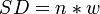$SD = n * w \,$

The standard deviation (SD) of the Gaussian representing any numerosity is that numerosity multiplied by the Weber fraction. Why is this the case? Intuitively, it is the standard deviations of the underlying Gaussian representations that determines the amount of overlap between the curves that represent any two numerosities, and it is the amount of overlap that determines how well any two numerosities can be discriminated.

The categorical views of the Weber fraction as a kind of threshold between successful discrimination and failure, or as the midpoint between subjective equality and asymptotic performance, choose to focus on only one particular point of what is actually a continuous and smooth function of increasing success at discrimination, and this entire function is determined by the Weber fraction as this fraction describes the standard deviation of any numerosity representation on the mental number line and thereby the degree of overlap between any two numerosities on the mental number line.

Viewed in this light, the Weber fraction describes all of the numerosity representations in the ANS. It is not specific to the task of discriminating two numerosities, and not specific to numerosity comparisons near “threshold”. In fact, though this may never be the case in practice, given this understanding of the Weber fraction it would be possible to assess the Weber fraction for an animal who could only represent a single number in the ANS (e.g., can only represent approximately-12). It would be the standard deviation of the Gaussian that represents approximately-12 divided by the mean of this Gaussian.

## The Weber Fraction as a Scaling Ratio

The Weber fraction (w) is the constant that describes the amount of variability in the underlying ANS number representations. It is a scaling factor by which one could take any one of the curves in Figure 1a and turn it into any of the other curves in Figure 1a. In the linear model in Figure 1a, the analog representation for any numerosity (e.g., n = 7) is a Gaussian random variable with a mean at n (e.g., n = 7) and a standard deviation of (n * w). This means that for a subject who has a Weber fraction of .125, the ANS representation for n = 7 will be a normal curve with a mean of 7 on the mental number line and a standard deviation of .125 * 7 = .875.

By substituting any number you like for n you can easily determine the shape of the underlying ANS representation, without ever having the participant engage in a numerical discrimination task that compares two numbers. This illustrates the power of understanding the Weber fraction as an index of internal noise: rather than simply telling us something about how well a subject will do at discriminating two numbers near their threshold, the Weber fraction (w) tells us the shape and overlap of every single number representation inside a person’s Approximate Number System (ANS). The Weber fraction is about all of the representations, not just the ones “near threshold.”

The inductive power of understanding the Weber fraction (w) to be an internal scaling factor is also seen when we compare the Weber fractions of different individuals. Individuals differ in the precision of their ANS representations. Some people have more noise and some people have less.

In Figure 4a I illustrate some idealized curves which represent the underlying ANS representations for a subject whose w = .125 and in Figure 4b for a subject whose w = .20. Crucially, one can see that the subject in Figure 4b has a greater degree of overlap between the Gaussian curves that represent numerosity than the subject in Figure 4a (recall, a bigger Weber fraction means more noise and fatter curves). It is this overlap that leads to difficulty in discriminating two stimuli that are close in numerosity (i.e., as one nears Weber Ratio = 1). The hypothetical subject in Figure 4b would have poorer discrimination in a numerical discrimination task (e.g., blue & yellow dots task) than the subject in Figure 4a.

In Figure 4c I have drawn the ideal performance for these two subjects in a numerical discrimination task. The midpoint between subjective equality (Percent Correct = 50%) and asymptotic performance (Percent Correct = 100%) in Figure 4c will fall at WR = 1.125 for the subject in Figure 4a and at WR = 1.20 for the subject in Figure 4b because these are equal to the Weber fraction for each subject plus one. An inspection of Figure 4c reveals that this is the case. This is not an accident; it derives from the relationship described above between the Weber fraction (w), the standard deviations of the underlying ANS number representations, and the overlap between any two ANS number representations.

The values for the Weber fractions in Figure 4 have been chosen so as to illustrate another value of understanding the Weber fraction to be an internal scaling factor; it empowers comparisons across individuals and formal models of individual differences. Converting the Weber fraction for each of these subjects into the nearest whole number fraction reveals that the Weber fraction for the subject in Figure 4a is 8:9 and for the subject in Figure 4b is 5:6 (i.e., 9 / 8 = 1.125; 6 / 5 = 1.20). Investigating the Gaussian curves in Figure 4a and 4b, which were created by treating the Weber fraction as an internal scaling factor to determine the standard deviations of the ANS Gaussian curves, reveals that the Gaussians for the numerosities 8 and 9 for the subject in Figure 4a are identical to the Gaussians for the numerosities 5 and 6 for the subject in Figure 4b. This is no accident. The only parameter that has been changed in constructing Figures 4a and 4b is the Weber fraction for the subject and this single parameter determines the spread in the Gaussians that represent every possible numerosity in the ANS of each subject.

In this way, understanding the Weber fraction to be an internal scaling factor that determines the spread of every ANS number representation not only empowers us to compare one number representation to another within a particular subject, it also empowers us to compare across individuals and to create mathematically tractable predictions about how the ANS representations of one person (e.g., the subject in Figure 4a) relate to the ANS representations of another (e.g., the subject in Figure 4b).

## Deviations From the Panamath Model

It is not uncommon for performance in a discrimination task to deviate from the ideal performance depicted in e.g., Figure 1b and seen in actual data in Figure 2a. Two basic deviations capture almost all cases: 1) a “give-up” function wherein subjects give-up on their numerical discrimination abilities on hard ratios and guess at chance, and 2) a “lapse parameter” which tracks participants’ tendency to have a lapse in attention such that they do not attend the e.g., blue and yellow dots on a particular trial. These two forms of deviation from the model presented in this chapter are tractable in that additional parameters can be included in the model to capture these behaviors.

The model I have described does not take account of participants’ confidence in their answers, nor does it adjust its predictions based on sub-optimal engagement of the Approximate Number System (ANS). But, in practice, sub-optimal performance can occur, especially on difficult trials.

For example, consider the task of being shown a brief display of blue and yellow dots and being asked to guess which color had more dots. One a fairly easy trial (e.g., 27 yellow versus 7 blue), you would most likely get the correct answer and be highly confident in your answer. This would be true even if the display time was incredibly brief (e.g., 50 msec).

## Effect of Difficulty on Trials

But, what will happen on a difficult trial of e.g., 27 yellow and 28 blue dots? On such a trial, a subject can tell fairly rapidly that the trial will be incredibly difficult and they will have very low confidence that their guess will be correct. On such trials, it is not unreasonable that a subject will simply give up on trying to determine whether there are more blue or more yellow dots and guess randomly (e.g., flip a coin). This kind of behavior has been observed in numerical discrimination tasks that present trials that are very difficult for that subject (e.g., presenting 3-year-olds with a brief display of 9 versus 10 objects) and it has also been observed in the discrimination performance of many animal models making a variety of ratio-dependent discriminations (but has yet to be discussed in detail in any literature). When this happens, performance will deviate from the model shown in Figure 1b in that percent correct will drop to chance before the Weber Ratio = 1. Participants will “give-up” on difficult trials and, rather than relying on their ANS representations, will guess randomly. This kind of performance can be seen in the left side of the curves diagramming the performance of children and adults in a difficult numerical discrimination task (Figure 5).

It is important to note that this kind of drop to chance performance is not predicted by any existing psychophysical model and it appears to be a deviation from the model caused by the subject giving up on the task; it does not appear to be the case, as of yet, that this type of deviation indicates that the underlying model of the ANS itself is flawed. We are currently attempting to specify the exact relationship between difficulty, Weber fraction, and the probability of giving up on a trial.

## Effect of Attention

Another deviation can occur on the right side of the psychometric curve and can also be seen in Figure 5. As has been observed in previous numerical discrimination tasks, performance in unskilled subjects can fail to reach 100% correct even on easy trials, perhaps because of a tendency to suffer a lapse of attention on a subset of trials. As can be seen in the example data in Figure 5, this was the case for the 3-, 4- and 5-year-olds.

When this type of lapse occurs, it should not be dependent on the ratio that was presented on that trial. Rather, it is an event that occurs because of e.g., fatigue, irrespective of the ratio being presented on that particular trial. As such, this deviation is typically detectable as a flat cut in percent correct across the entire range of ratios presented in an experiment. It is because percent correct is highest on the easiest ratios that the deleterious affects of this lapse in attention are most visible on easier trials. The simplest way to account for this tendency in the model is to include a lapse parameter that is a constant probability of guessing randomly on any particular trial.

This parameter primarily lowers the model’s asymptotic performance while retaining an accurate estimate of the Weber fraction (w). When required, I included this parameter in the model as follows, where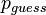$p_{guess} \,$ is the probability of guessing randomly on any particular trial irrespective of ratio,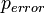$p_{error} \,$ is the probability of being incorrect given the model above and the chance level is .5 multiplied by 100 to return a percentage: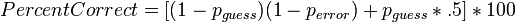$Percent Correct = [(1 - p_{guess} )(1 - p_{error} ) + p_{guess} * .5 ] * 100 \,$

This type of guessing has also been observed in children (Figure 5) and in animal and other adult human participants for both numerical discrimination and other ratio-dependent dimensions.

## What Do These Deviations Mean?

Each of these deviations from the model presented in this chapter can be captured by including an additional parameter in the model (i.e., a threshold for “giving up” as a function of ratio, and a lapse parameter; the probability of guessing randomly on any particular trial irrespective of ratio).

At the moment, the existing datasets do not suggest that deviation from the model presented here is so drastic as to recommend the abandoning of the model. Rather, as with all complex cognition, behaviors that engage the Approximate Number System (ANS) are occurring in a rich context that drives many other considerations that can affect performance. Giving up on a hard trial and being bored such that you fail to attend on a trial are two very basic considerations that can affect performance.

## Conclusions and Summary

If one does some reading in hopes of understanding what a Weber fraction (w) is, the most common descriptions turn on the idea of a difference threshold (aka Just Noticeable Difference, J.N.D.). For example, “the fraction by which a stimulus with numerosity n would need to be increased in order for a subject to detect this change and its direction on 50% of the trials” is a common phrase; or, “the midpoint that indicates roughly successful discrimination performance.”

Thinking about a Weber fraction in these ways promotes confusions and limits our inferences (e.g., the confusion that performance should change from chance performance at difficult ratios to above chance performance at easy ratios; as we’ve seen in Figure 3, the actual performance of subjects does not look this way but instead is a smooth function). It also does not promote the kind of understanding of the Approximate Number System (ANS) that highlights the systematic nature of the noise across various ANS number representations within an individual (e.g., understanding that the noise for any one ANS representation can be easily translated into an understanding of the noise for every single ANS number representation), nor the systematic relationships that exist across individuals (e.g., the comparison of the two subjects in Figure 4).

In this chapter I have tried to promote a third way of understanding the Weber fraction: it is a scaling factor that enables any ANS number representation to be turned into any other; or, equivalently, it is an index of the amount of noise in every one of the Approximate Number System (ANS) representations. Understood in this way, a Weber fraction does not require the commonsense notion of a “threshold” (i.e., a change from failure to success) and does not generate the same kinds of confusions that this commonsense notion gives rise to.

The commonsense notion of a “threshold,” as a change from poor to good performance, is a productive one for first coming to understand how discrimination behavior works across many dimensions (e.g., approximate number, loudness, brightness etc). And so, it should not be abandoned. But it does not foster our intuitions about the underlying representations that give rise to this discrimination behavior (i.e., it doesn’t give rise to an accurate picture of the systematic relationships between noise, numerosity, and discrimination performance). An understanding of a Weber fraction (w) as an index of the internal noise in the underlying ANS representations promotes productive intuitions and better theorizing about the Approximate Number System (ANS) and other ratio-dependent cognitive dimensions (e.g., line length, weight, brightness, etc).

A Weber fraction (w) indexes the amount of noise in the underlying approximate number representations, it specifies the standard deviations of the underlying Gaussian number curves for every numerosity in a subject’s Approximate Numer System (ANS) and thereby the degree of overlap between any of these representations. For discrimination, the Weber fraction describes a subject’s performance at discriminating any possible combination of numerosities, not just discriminability near their “discrimination threshold”. And, the Weber fraction (w), understood as an internal scaling factor, describes all of the representations in the Approximate Number System (ANS) without any reference to a numerical discrimination task.